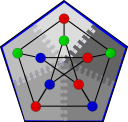#Boost C++ Libraries

...one of the most highly regarded and expertly designed C++ library projects in the world.

#s-t Connectivity

```namespace graph { namespace distributed {
template<typename DistributedGraph, typename ColorMap>
inline bool
st_connected(const DistributedGraph& g,
typename graph_traits<DistributedGraph>::vertex_descriptor s,
typename graph_traits<DistributedGraph>::vertex_descriptor t,
ColorMap color)

template<typename DistributedGraph>
inline bool
st_connected(const DistributedGraph& g,
typename graph_traits<DistributedGraph>::vertex_descriptor s,
typename graph_traits<DistributedGraph>::vertex_descriptor t)

template<typename DistributedGraph, typename ColorMap, typename OwnerMap>
bool
st_connected(const DistributedGraph& g,
typename graph_traits<DistributedGraph>::vertex_descriptor s,
typename graph_traits<DistributedGraph>::vertex_descriptor t,
ColorMap color, OwnerMap owner)
} }
```

The st_connected() function determines whether there exists a path from s to t in a graph g. If a path exists the function returns true, otherwise it returns false.

This algorithm is currently level-synchronized, meaning that all vertices in a given level of the search tree will be processed (potentially in parallel) before any vertices from a successive level in the tree are processed. This is not necessary for the correctness of the algorithm but rather is an implementation detail. This algorithm could be rewritten fully-asynchronously using triggers which would remove the level-synchronized behavior.

# Where Defined

<boost/graph/distributed/st_connected.hpp>

# Parameters

IN: const DistributedGraph& g
The graph type must be a model of Distributed Graph. The graph type must also model the Incidence Graph.

IN: vertex_descriptor s

IN: vertex_descriptor t

UTIL/OUT: color_map(ColorMap color)
The color map must be a Distributed Property Map with the same process group as the graph g whose colors must monotonically darken (white -> gray/green -> black). The default value is a distributed two_bit_color_map.
IN: OwnerMap owner
The owner map must map from vertices to the process that owns them as described in the GlobalDescriptor concept.
OUT: bool
The algorithm returns true if a path from s to t is found, and false otherwise.

# Complexity

This algorithm performs O(V + E) work in d/2 + 1 BSP supersteps, where d is the shortest distance from s to t. Over all supersteps, O(E) messages of constant size will be transmitted.

# Algorithm Description

The algorithm consists of two simultaneous breadth-first traversals from s and t respectively. The algorithm utilizes a single queue for both traversals with the traversal from s being denoted by a gray entry in the color map and the traversal from t being denoted by a green entry. The traversal method is similar to breadth-first search except that no visitor event points are called. When any process detects a collision in the two traversals (by attempting to set a gray vertex to green or vice-versa), it signals all processes to terminate on the next superstep and all processes return true. If the queues on all processes are empty and no collision is detected then all processes terminate and return false.

Copyright (C) 2009 The Trustees of Indiana University.

Authors: Nick Edmonds and Andrew Lumsdaine